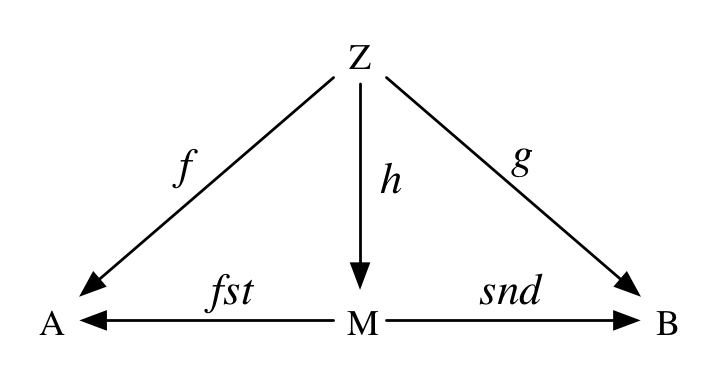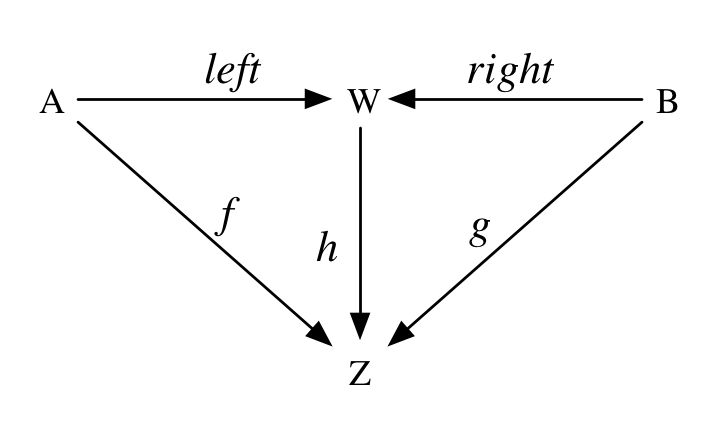# Monoid Morphisms, Products, and Coproducts

Today I want to talk about relationships between monoids. These can be useful to think about when we’re developing libraries involving monoids, and we want to express some algebraic laws among them. We can then check these with automated tests, or indeed prove them with algebraic reasoning.

This post kind of fell together when writing notes on chapter 10, “Monoids”, of Functional Programming in Scala. I am putting it here so I can reference it from the chapter notes at the end of the book.

## Monoid homomorphisms

Let’s take the `String` concatenation and `Int` addition as example monoids that have a relationship. Note that if we take the length of two strings and add them up, this is the same as concatenating those two strings and taking the length of the combined string:

So every `String` maps to a corresponding `Int` (its length), and every concatenation of strings maps to the addition of corresponding lengths.

The `length` function maps from `String` to `Int` while preserving the monoid structure. Such a function, that maps from one monoid to another in such a preserving way, is called a monoid homomorphism. In general, for monoids `M` and `N`, a homomorphism `f: M => N`, and all values `x:M`, `y:M`, the following equations hold:

The `|+|` syntax is from Scalaz and is obtained by importing `scalaz.syntax.monoid._`. It just references the `append` method on the `Monoid[T]` instance, where `T` is the type of the arguments. The `mzero[T]` function is also from that same import and references `zero` in `Monoid[T]`.

This homomorphism law can have real practical benefits. Imagine for example a “result set” monoid that tracks the locations of a particular set of records in a database or file. This could be as simple as a `Set` of locations. Concatenating several thousand files and then proceeding to search through them is going to be much slower than searching through the files individually and then concatenating the result sets. Particularly since we can potentially search the files in parallel. A good automated test for our result set monoid would be that it admits a homomorphism from the data file monoid.

## Monoid isomorphisms

Sometimes there will be a homomorphism in both directions between two monoids. If these are inverses of one another, then this kind of relationship is called a monoid isomorphism and we say that the two monoids are isomorphic. More precisely, we will have two monoids `A` and `B`, and homomorphisms `f: A => B` and `g: B => A`. If `f(g(b)) == b` and `g(f(a)) == a`, for all `a:A` and `b:B` then `f` and `g` form an isomorphism.

For example, the `String` and `List[Char]` monoids with concatenation are isomorphic. We can convert a `String` to a `List[Char]`, preserving the monoid structure, and go back again to the exact same `String` we started with. This is also true in the inverse direction, so the isomorphism holds.

Other examples include (`Boolean`, `&&`) and (`Boolean`, `||`) which are isomorphic via `not`.

Note that there are monoids with homomorphisms in both directions between them that nevertheless are not isomorphic. For example, (`Int`, `*`) and (`Int`, `+`). These are homomorphic to one another, but not isomorphic (thanks, Robbie Gates).

## Monoid products and coproducts

If `A` and `B` are monoids, then `(A,B)` is certainly a monoid, called their product:

But is there such a thing as a monoid coproduct? Could we just use `Either[A,B]` for monoids `A` and `B`? What would be the `zero` of such a monoid? And what would be the value of `Left(a) |+| Right(b)`? We could certainly choose an arbitrary rule, and we may even be able to satisfy the monoid laws, but would that mean we have a monoid coproduct?

To answer this, we need to know the precise meaning of product and coproduct. These come straight from Wikipedia, with a little help from Cale Gibbard.

A product `M` of two monoids `A` and `B` is a monoid such that there exist homomorphisms `fst: M => A`, `snd: M => B`, and for any monoid `Z` and morphisms `f: Z => A` and `g: Z => B` there has to be a unique homomorphism `h: Z => M` such that `fst(h(z)) == f(z)` and `snd(h(z)) == g(z)` for all `z:Z`. In other words, the following diagram must commute:A coproduct `W` of two monoids `A` and `B` is the same except the arrows are reversed. It’s a monoid such that there exist homomorphisms `left: A => W`, `right: B => W`, and for any monoid `Z` and morphisms `f: A => Z` and `g: B => Z` there has to be a unique homomorphism `h: W => Z` such that `h(left(a)) == f(a)` and `h(right(b)) == g(b)` for all `a:A` and all `b:B`. In other words, the following diagram must commute:We can easily show that our `productMonoid` above really is a monoid product. The homomorphisms are the methods `_1` and `_2` on `Tuple2`. They simply map every element of `(A,B)` to a corresponding element in `A` and `B`. The monoid structure is preserved because:

And for any other monoid `Z`, and morphisms `f: Z => A` and `g: Z => B`, we can construct a unique morphism from `Z` to `(A,B)`:

And this really is a homomorphism because we just inherit the homomorphism law from `f` and `g`.

What does a coproduct then look like? Well, it’s going to be a type `C[A,B]` together with an instance `coproduct[A:Monoid,B:Monoid]:Monoid[C[A,B]]`. It will be equipped with two monoid homomorphisms, `left: A => C[A,B]` and `right: B => C[A,B]` that satisfy the following (according to the monoid homomorphism law):

And additionally, for any other monoid `Z` and homomorphisms `f: A => Z` and `g: B => Z` we must be able to construct a unique homomorphism from `C[A,B]` to `Z`:

Right off the bat, we know some things that definitely won’t work. Just using `Either` is a non-starter because there’s no well-defined `zero` for it, and there’s no way of appending a `Left` to a `Right`. But what if we just added that structure?

### Free monoids on coproducts

The underlying set of a monoid `A` is just the type `A` without the monoid structure. The coproduct of types `A` and `B` is the type `Either[A,B]`. Having “forgotten” the monoid structure of both `A` and `B`, we can recover it by generating a free monoid on `Either[A,B]`, which is just `List[Either[A,B]]`. The `append` operation of this monoid is list concatenation, and the identity for it is the empty list.

Clearly `List[Either[A,B]]` is a monoid, but does it permit a homomorphism from both monoids `A` and `B`? If so, then the following properties should hold:

They clearly do not hold! The lists on the left of `==` will have two elements and the lists on the right will have one element. Can we do something about this?

Well, the fact is that `List[Either[A,B]]` is not exactly the monoid coproduct of `A` and `B`. It’s still “too big”. The problem is that we can observe the internal structure of expressions.

What we need is not exactly the `List` monoid, but a new monoid called the free monoid product:

`Eithers[A,B]` is a kind of `List[Either[A,B]]` that has been normalized so that consecutive `A`s and consecutive `B`s have been collapsed using their respective monoids. So it will contain alternating `A` and `B` values.

The only remaining problem is that a list full of identities is not exactly the same as the empty list. Remember the unit part of the homomorphism law:

This doesn’t hold at the moment. As Cale Gibbard points out in the comments below, `Eithers` is really the free monoid on the coproduct of semigroups `A` and `B`.

We could check each element as part of the normalization step to see if it `equals(zero)` for the given monoid. But that’s a problem, as there are lots of monoids for which we can’t write an `equals` method. For example, for the `Int => Int` monoid (with composition), we must make use of a notion like extensional equality, which we can’t reasonably write in Scala.

So what we have to do is sort of wave our hands and say that equality on `Eithers[A,B]` is defined as whatever notion of equality we have for `A` and `B` respectively, with the rule that `es.fold[(A,B)]` defines the equality of `Eithers[A,B]`. For example, for monoids that really can have `Equal` instances:

So we have to settle with a list full of zeroes being “morally equivalent” to an empty list. The difference is observable in e.g. the time it takes to traverse the list.

Setting that issue aside, `Eithers` is a monoid coproduct because it permits monoid homomorphisms from `A` and `B`:

And `fold` really is a homomorphism, and we can prove it by case analysis. Here’s the law again:

If either of `e1` or `e2` is empty then the result is the fold of the other, so those cases are trivial. If they are both nonempty, then they will have one of these forms:

In the first two cases, on the right of the `==` sign in the law, we perform `a1 |+| a2` and `b1 |+| b2` respectively before concatenating. In the other two cases we simply concatenate the lists. The `++` method on `Eithers` takes care of doing this correctly for us. On the left of the `==` sign we fold the lists individually and they will be alternating applications of `f` and `g`. So then this law amounts to the fact that `f(a1 |+| a2) == f(a1) |+| f(a2)` in the first case, and the same for `g` in the second case. In the latter two cases this amounts to a homomorphism on `List`. So as long as `f` and `g` are homomorphisms, so is `_.fold(f,g)`. Therefore, `Eithers[A,B]` is a coproduct of `A` and `B`.